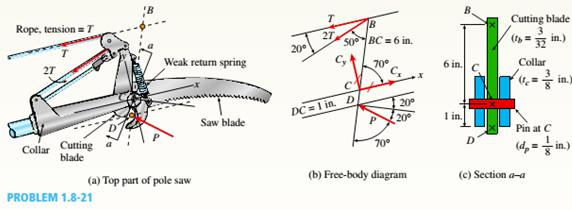# The top portion of a pole saw used to trim (a) Find the force P on the cutting Made at D if tbe small branches from trees is shown in the figure tension force in the rope is T = 25 lb (see free- part a. The cutting blade BCD (see figure parts a and body diagram in figure part b). c) applies a force P at point D. Ignore the effect of (b) Find force in the pin at C. the weak return spring attached to the cutting blade (c) Find average shear stress r a v e r and bearing below B. Use properties and dimensions given in the stress in the support pin at C (see section a-a figure. through cutting blade in figure part c).### Mechanics of Materials (MindTap Co...

9th Edition
Barry J. Goodno + 1 other
Publisher: Cengage Learning
ISBN: 9781337093347### Mechanics of Materials (MindTap Co...

9th Edition
Barry J. Goodno + 1 other
Publisher: Cengage Learning
ISBN: 9781337093347
Chapter 1, Problem 1.8.21P
Textbook Problem
3 views

## The top portion of a pole saw used to trim (a) Find the force P on the cutting Made at D if tbe small branches from trees is shown in the figure tension force in the rope is T = 25 lb (see free-part a. The cutting blade BCD (see figure parts a and body diagram in figure part b).c) applies a force P at point D. Ignore the effect of (b) Find force in the pin at C.the weak return spring attached to the cutting blade (c) Find average shear stress raver and bearing below B. Use properties and dimensions given in the stress in the support pin at C (see section a-afigure. through cutting blade in figure part c).(a)

To determine

The force on the cutting plate at D, if the tension force in the rope is given.

### Explanation of Solution

Given Information:

Tension T = 25 lb.

Thickness of the cutting blade tb=332in. and,

Thickness of collar tc=38in.

Diameter of pin dp=18in.

Diameter of dbc=6in.

Diameter of dcd=1in.

(b)

To determine

The force in the pin at C.

(c)

To determine

The average shear stress τ and bearing stress σb in the support pin at C.

### Still sussing out bartleby?

Check out a sample textbook solution.

See a sample solution

#### The Solution to Your Study Problems

Bartleby provides explanations to thousands of textbook problems written by our experts, many with advanced degrees!

Get Started

Find more solutions based on key concepts
What are the two major types of cutting fluids?

Precision Machining Technology (MindTap Course List)

What are the basic characteristic of a linear model?

Engineering Fundamentals: An Introduction to Engineering (MindTap Course List)

Determine the largest force P for which the 16-kg uniform bar remains in equilibrium.

International Edition---engineering Mechanics: Statics, 4th Edition

Security Secure IT 1-3 in this chapter offers advice about creating secure passwords when registering for websi...

Enhanced Discovering Computers 2017 (Shelly Cashman Series) (MindTap Course List)

How can discontinuities in the root face be removed?

Welding: Principles and Applications (MindTap Course List)

If your motherboard supports ECC DDR3 memory, can you substitute non-ECC DDR3 memory?

A+ Guide to Hardware (Standalone Book) (MindTap Course List)